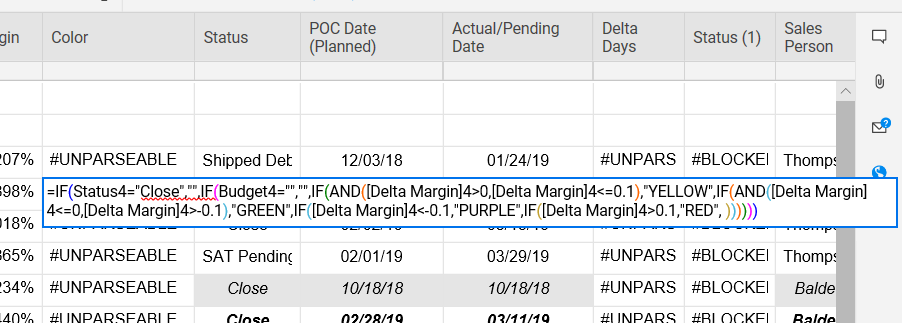# Importing excel IF/And statments to Smartsheets

I use the below IF/AND statement in excel.  It works fine.

=IF(O4="Close","",IF(D4="","",IF(AND(M4>0,M4<=0.1),"YELLOW",IF(AND(M4<=0,M4>-0.1),"GREEN",IF(M4<-0.1,"PURPLE",IF(M4>0.1,"RED",))))))

When I import it into Smartsheets, the formula is below and doesn't work.  I get #UNPARSEABLE in the cell.  Does anybody know why the formula is not working?

=IF(Status3="Close","",IF(Budget3="","",IF(AND([Delta Margin]3>0,[Delta Margin]3<=0.1),"YELLOW",IF(AND([Delta Margin]3<=0,[Delta Margin]3>-0.1),"GREEN",IF([Delta Margin]3<-0.1,"PURPLE",IF([Delta Margin]3>0.1,"RED", ))))))

Best Regards,• The first thing I see is the end. In smartsheet if there is a comma the program expects a return. Try adding "" after the last comma.

Also quick note for troubleshooting these stacked if statements, try deleting all of the closing parenthesis at the end of the statement then hit enter. Smartsheet will calculate how many it needs, and put them there for you if your formula is correct.

• Thanks for the suggestion but it didn't work.  Any more thoughts?

Thanks

• =IF(Status3 = "Close", "", IF(Budget3 = "", "", IF(AND([Delta Margin]3 > 0, [Delta Margin]3 <= 0.1), "YELLOW", IF(AND([Delta Margin]3 <= 0, [Delta Margin]3 > -0.1), "GREEN", IF([Delta Margin]3 < -0.1, "PURPLE", IF([Delta Margin]3 > 0.1, "RED"))))))

I spaced it out so it is easier for me to read, but other than that the only change I made was to remove the last comma and following space, and your closing parenthesis are correct as is the syntax.

Other than that...

Because the parenthesis in your screenshot are colored but the cell references are not, it leads me to believe there may be a typo in the cell references. Double check your column names.

• Yep, that worked.  Thank you very much for your assistance.

Best Regards,